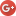## Aleksandr V. Bukalove-mail: boukalov@gmail.com
The Centre of Physical and Space Researches, International Institute of Socionics
"Physics of Consciousness and Life, Cosmology and Astrophysics" journal, issue 1, 2002 .

# The modes of evolution of the quantum universe

For the description of the quantum universe evolution it has been suggested an equation, which particular case is the equation of the dynamics of the inflationary universe. It has been considered a possible sequence of the four modes of the quantum universe.
Key words: quantum universe, inflationary universe.

For the linear damped harmonic oscillation the equation of motion is the following :,   (1)
where θ is quality, 2θ=ωτ, τ is the character time of the oscillation damping.

Equation (1) from the classic theory of oscillations completely coincides with the equations, which describe the inflationary universe, that is filled in by the scalar field φ [2, 3]:.   (2)

Let us note also that if θ-1=3, then 2θ = ωτ =2/3. In that case the time of the oscillator damping is
τ =2/3ω0=2/3H   (3)

However, taking into account the influence on the oscillations of the positive and negative feed-back, connected with the dynamic of the evolution of vacuum fields, it is necessary to use the equation,   (4)
when a is the parameter of the connection: if a>0, then it is the positive feed-back, if a<0, then it is the negative feed-back.

Equation (4) is equivalent to equation for the damped or exited harmonic oscillator with the modified quality θ*=θ(1-a)-1:,    (5)
that is equivalent for the inflationary theory of the universe to the equation(6)

The influence of the positive and negative feed-back is described with the use of the modified quality, which is shown on Fig. 1

From this, more common point of view, the theory of the inflationary universe is a particular case of the oscillations of the φ-field, or the universe as a whole, described by equation (6).

The existence of the other modes, which the universe has to give in the process of its evolution, follows from (5) and Fig. 1. In this case the universe in the process of its development can pass, apparently, all modes with θ*-1(-∞;+∞) and a(+∞;-∞). That time θ*-1≤0 (a≥1), but the concrete values of θ*-1 and a will be made more precise by the further experimental and theoretical researches.References:

1. Bukalov A.V. On the cosmological triadic structure of Metagalaxy as the psycho-informational system. // Physics of Consciousness and Life, Cosmology and Astrophysics. — 2001. — ą 3.
2. Dolgov A.D., Zel'dovic J.B., Sagin M.V. Kosmologija rannej Vselennoj. — Moskow. 1988. — 199 p.
3. Linde A.D. Particle Physics and Inflationary Cosmology. — Boston, MA: Harvard University Press, 1990.
4. Guth A.H., 1981, Phys.Rev. D, 23, 347.
5. Freedman W., 1999. Preprint astro-ph/9909076.
6. Kneubühl F.K. Repertitorium der Physik. — B.G.Teubner. Stuttgart, 1975.